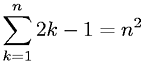Equations > Calculus > Sequences and Series > Arithmetic Series - Sequential Odd Integers

### Arithmetic Series - Sequential Odd IntegersLatex Code:

MathML Code:

 $\sum _{k=1}^{n}2k-1={n}^{2}$

MathType 5.0: